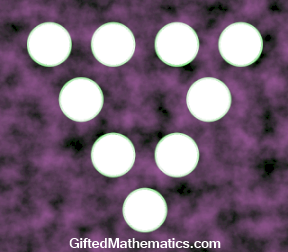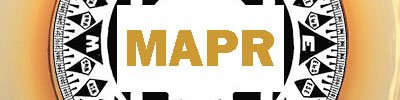# Brainsteem: Primes in a Triangle - Prize Tokens!

in #mathematics3 years ago

It's been a very long time since I last posted a Brainsteem mathematical puzzle. Under some prompting from @quantumdeveloper, followed by a bit of procrastination, I've finally got round to posting.

The problem with this problem is that the most obvious solution algorithm does give an answer, but it isn't the minimal answer requested!

So perhaps with some fresh minds on the case, this time will be different.

Contests need prizes, so the the prize will be one MAP FinTech token to the first correct answer plus one more token to either a random draw of other answers or to a comment of particular interest. If this latter sounds vague, then that's because it's meant to be - if it's interesting, and relevant, then it may be rewarded.

The Question

The diagram below shows a triangle constructed of four circles along each side.

Insert a different prime number within each circle in such a way so that the sums of the four numbers along each side are all equal.What is the smallest possible sum of all nine primes?

The Prize

One MAPR token to the first correct answer plus one extra MAPR token to any interesting comments!

Building Steem's FintechSort:

I get to 153 by placing them like this(clockwise starting in bottom):
`3, 11, 37, 5, 13, 31, 7, 17, 29`
But I guess that was the obvious algorithm, right?

Correct! I mean... right!
;-)
There is a smaller solution.

Since the obvious algorithm didn't work I decided to brute force through the first 20 primes except 2.
The result was(clockwise, starting at the bottom):
`127 with 3, 17, 23, 7, 11, 19, 13, 5, 29`
That are the first 9 primes after 2, so I guess I should have reached that without my computer.

I was curious and tested a bit further and the triangle seems to work for any sequence of 9 consecutive primes (that I tested). Is that a global fact or is it just valid for the first few cases? If yes, why?

So your corners are 3, 7 and 13 and each edge adds to 50.
That's funny, coz better than the one I got by hand!

I thought it would have something to do with prime-pairs, but with nine numbers it means at least one of them must be outside that simple rule.

How far up did you test this?

If we take the middle number as p, (p=13 in this case) then the other numbers are:
p+4, p+6, p+10, p+16,
p-2, p-6, p-8, p-10.
The sum is then 9p+10.

Is the median always on a corner?

3 years ago (edited)

I only tested the first few cases. Further testing revealed it only works for the first 35 prime sequences.

The median is only for 200 out of 700 cases I tested on one of the corners.

You found 700 solutions... so far?

So what's the largest sum so far?

Would a sequence of the prime sums terminate?

:-)

No… I found 44442 solutions.
The highest one is:
`11696519: {1299541, 1299653, 1299673, 1299553, 1299583, 1299637, 1299647, 1299601, 1299631};`

If you are interested in the other solutions(with only consecutive primes(generating a sequence containing also all non-consecutive-prime solutions would take ages with brute force)) you can find a text document with all 44442 of them here

I don't think there would an end in the sequence. With infinitely many primes there should be a new number in the sequence when you search through enough primes.
However the sequence with consecutive primes only might end at some point, but it would certainly be after much more then 44442.

Update: I let the program run even further. I now got 351166 solutions.
The highest one is:
`139372413: {15485807, 15485801, 15485857, 15485837, 15485773, 15485843, 15485849, 15485783, 15485863};`

This starts to look like prime k-tuplets; in our case 9-tuplets.You distributed more than 58000 upvotes. Your next target is to reach 59000 upvotes.
If you no longer want to receive notifications, reply to this comment with the word `STOP`